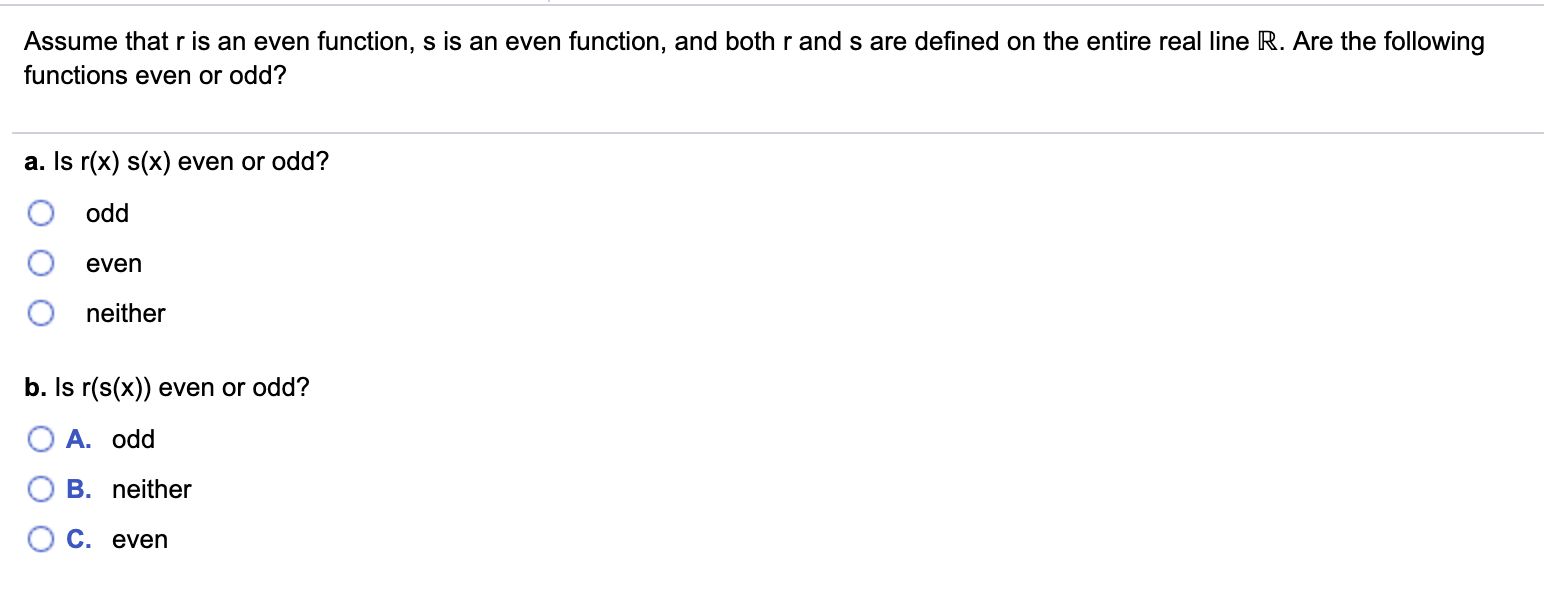Home / Answered Questions / Other / assume-that-r-is-an-even-function-s-is-an-even-function-and-both-r-and-s-are-defined-on-the-entire-r-aw968

# (Solved): Assume That R Is An Even Function, S Is An Even Function, And Both R And S Are Defined On The Entire...Assume that r is an even function, s is an even function, and both r and s are defined on the entire real line R. Are the following functions even or odd? a. Is r(x) s(x) even or odd? O odd O even neither b. Is r(s(x)) even or odd? O A. odd O B. neither O c. even

We have an Answer from Expert